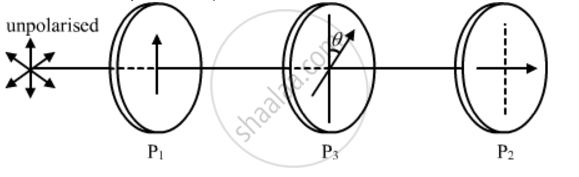# Two Polaroids P1 and P2 Are Placed with Their Pass Axes Perpendicular to Each Other. an Unpolarised Light of Intensity Io is Incident on P1. - Physics

Two polaroids P1 and P2 are placed with their pass axes perpendicular to each other. An unpolarised light of intensity Io is incident on P1. A third polaroid P3 is kept in between P1 and P2 such that its pass axis makes an angle of 45° with that of P1. Determine the intensity of light transmitted through P1, P2 and P3

#### Solution

As given in the question, the polaroids P1 and P2 are placed with their pass axes perpendicular to each other. Also, P3 is placed at an angle of 45° with respect to P1.Now, we have:

Intensity of light after falling on PI'=I_0/2

Intensity of light after falling on P3 I"=

I'cos^2(theta)=I_0/2cos^2(45^@)=I_0/4

Therefore, a light of intensity I_0/4will pass through P3 and the angle between P3 and P2 will be 45° because of the condition given in the question.

Intensity of light after falling on PI''' = I'cos^2(theta)=I_0/4cos^2(45^@)=I_0/8

Concept: Polarisation
Is there an error in this question or solution?
2013-2014 (March) All India Set 2

Share